# SAS/ETS Examples

## Bootstrapping Correct Critical Values in Tests for Structural Change

Contents | SAS Program

# Overview

Tests for structural change in a time series variable are typically performed by modeling a likely breakpoint for the structural model with a dummy variable that has value 0 before the break and value 1 after the break. The residual sum of squares from this unrestricted model and a restricted model with no breakpoint are compared using the standard F -test.

Christiano, in his 1988 paper "Searching for a Break in GNP," questioned this traditional method of testing for structural change in a time series process. The gist of his paper is that traditional methods of testing for a break in trend are flawed in that the significance levels overstate the likelihood of the trend break alternative hypothesis. He attributes this to the fact that conventional tests assume that the break point is exogenous to the test, whereas, in practice, the break point is almost always chosen a priori.

Christiano uses quarterly data on log GNP from 1948:1 to 1988:4 to fit the trend-stationary (TS) model
A battery of F-tests is computed for each of the draws from the error distribution. The unrestricted model is
where
for i = 3, ... , T-2. The dummy variable dt has value zero before the break point i and a value of one after break point i.

The critical values come from the 95th percentile of each row of the following matrix: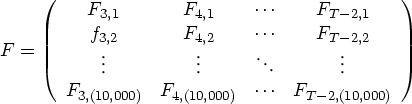where Fij is the F-value for the ith breakpoint in the jth simulation.

Pre-test adjusted critical values take into account the fact that the break point is commonly picked before the test is computed. To account for this bias, the 95th percentile of the largest F-value from each simulation is calculated.

# Analysis

The data for this example comes from the Citibase data set CITIQTR in the SASHELP library. The variables DATE, GDPQ, and L_GDPQ are read into the SAS DATA set GDP, where GDPQ is the quarterly gross domestic product in 1987 dollars from 19081:1 to 1991:4. L_GDPQ is the logarithmic transformation of GDPQ.

   data gdp;
set sashelp.citiqtr;
keep date gdpq l_gdpq;
l_gdpq = log(gdpq);
run;


A plot of the data in Figure 1 reveals a likely candidate for a structural break around 1983.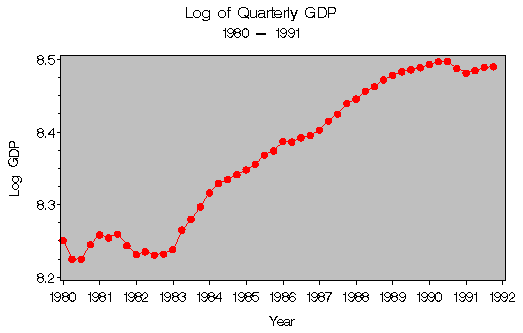Figure 1: Log of Quarterly GDP,1980 - 1991

SAS's Interactive Matrix Language (SAS/IML) is used to perform the 10,000 simulations for this bootstrapping example. IML is a powerful language that enables you to read variables from SAS DATA sets into matrices for computations and manipulations that may be too complicated or unwieldy in traditional DATA steps or procedures.

Begin by invoking PROC IML, reading the time series of interest, and initializing a few variables.

   proc iml;

simnum = 10000;
echo   = 500;

use gdp;
ldata = log(data);

nlag = 2;
strtvls = ldata[1:nlag,];

y    = ldata[3:nrow(ldata)];
y_1  = ldata[2:nrow(ldata)-1];
y_2  = ldata[1:nrow(ldata)-2];


SIMNUM sets the number of simulations to be performed equal to 10,000; ECHO is a feedback variable that is used later in the program to give you information on the progress of the simulation. In this case, at every 500 observations the program will print the total number of simulations computed to that point. Y, Y_1, and Y_2 are vectors corresponding to the time series variable GDPQ and its two lagged variables.

The next step is to define some modules which can be thought of as either functions or subroutines.

The module BATTERY takes as input the time series variables Y, Y_1, and Y_2 and returns as output a column vector F-tests, one for each possible breakpoint. The module also returns the matrix of variables in the restricted model and the number of variables in the restricted and unrestricted models. Notice that the dummy variable DI is used to control the breakpoint. The F-values are calculated directly using matrix algebra.

   start battery(y,y_1,y_2) global(xr, k, m);
batf = 0;
n    = nrow(y);
t    = cusum(j(n,1,1));
xr   = j(n,1,1) || t || y_1 || y_2;
m    = ncol(xr);
er   = (i(n) - xr*inv(xr*xr)*xr)*y;
do i=(m-1) to n-2 by 1;
di    = j(i-1,1,0) // j(n-i+1,1,1);
xu    = j(n,1,1) || di || t || di#t || y_1 || y_2;
k     = ncol(xu);
eu    = (i(n) - xu*inv(xu*xu)*xu)*y;
uss   = eu*eu;
batf  = batf // fstat;
end;
batf = batf[2:nrow(batf),];
return(batf);
finish battery;


The module BOOTSTRP takes as input the number of observations in the original data set NDATA, a vector of starting values, STRTVLS, a vector of fitted errors from which to make random draws EHAT, and the vector of parameter estimates BETAHAT from the fitted model. It returns a column vector YSIM, of simulated data.

   start bootstrp(ndata, strtvls, ehat, betahat);
ner     = nrow(ehat);
rndehat = j(ner,1,1);
do eloop=1 to ner by 1;
rndehat[eloop] = ehat[ceil(ner*uniform(0))];
end;
ysim    = j(ndata,1,1);
ysim[1:nrow(strtvls),] = strtvls;
t       = cusum(j(ndata,1,1));
do l=(nrow(strtvls)+1) to ndata by 1;
ysim[l] = betahat +
betahat*t[l-2] +
betahat*ysim[l-1] +
betahat*ysim[l-2] +
rndehat[l-2];
end;
return(ysim);
finish bootstrp;


The module SORTCM sorts each row of the input matrix by column and returns the resultant sorted matrix.

   start sortcm(x);
temp1=x;
do i=1 to nrow(temp1) by 1;
temp2 = temp1[i,];
temp3 = temp2;
temp2[,rank(temp2)] = temp3;
temp1[i,] = temp2;
end;
return(temp1);
finish sortcm;


The main program begins by computing a batter of F-tests for the original data and calculating the parameter estimates and residuals from the restricted TS model.

   fdata   = battery(y, y_1, y_2);

temp    = cusum(j(nrow(fdata)+m-2,1,1));
brkpt   = temp[(m-1):nrow(temp),];
ehat    = (i(nrow(y)) - xr*inv(xr*xr)*xr)*y;
betahat = inv(xr*xr)*xr*y;


Next, the F matrix is initialized and the 10,000 simulations performed. The results of each simulation are horizontally concatenated to the F matrix and the current simulation number is printed on the screen every 500 iterations.

   f     = j(nrow(ehat)-2*(k-m),1,0);
ndata = nrow(ldata);

do simloop=1 to simnum by 1;
ysim = bootstrp(ndata, strtvls, ehat, betahat);
y   = ysim[3:ndata];
y_1 = ysim[2:ndata-1];
y_2 = ysim[1:ndata-2];
f = f || battery(y, y_1, y_2);
if mod(simloop,echo)=0 then print simloop;
end;


The maximum F statistic for each simulation is found and sorted in the row vector FCOLMAX. The rows of F are sorted by column to create the matrix FSORT. The column associated with the 95th percentile is selected for each matrix as well as a column vector of the 95% critical value for the standard F-test, becoming the variables F_MAX, F_95, and F_STD, respectively.

   f       = f[,2:ncol(f)];
fcolmax = sortcm(f[<>,]);
fsort   = sortcm(f);
cv95    = int(.95*simnum);

brkpt   = date[brkpt,];
fdata   = fdata;
fstd_95 = j(nrow(fdata),1,finv(.95,k-m,nrow(ehat)-k));
f_95    = fsort[,cv95];
fmax_95 = j(nrow(fdata),1,fcolmax[,cv95]);


Finally, a SAS DATA set is created containing the variables of interest and PROC IML is exited.

   create sasuser.bootout
var{brkpt fdata fstd_95 f_95 fmax_95};
append;

quit;


The GPLOT procedure can be used to view the results of the test, shown in Figure 2.

   title 'Bootstrapped Critical Values';

axis1 label=('Break Point') minor=none
order=('01jan80'd to '01jan91'd by year);
axis2 label=(angle=90 'F-statistics')
order=(0 to 15 by 3);

symbol1 c=red   i=join;    /* for fdata   */
symbol2 c=black i=spline;  /* for fstd_95 */
symbol3 c=green i=spline;  /* for f_95    */
symbol4 c=blue  i=spline;  /* for fmax_95 */

proc gplot data=sasuser.bootout;
format brkpt year4.;
plot fdata   * brkpt = 1
fstd_95 * brkpt = 2
f_95    * brkpt = 3
fmax_95 * brkpt = 4 / overlay
vaxis=axis2
vminor=1
haxis=axis1
legend
cframe=ligr;
run;
quit;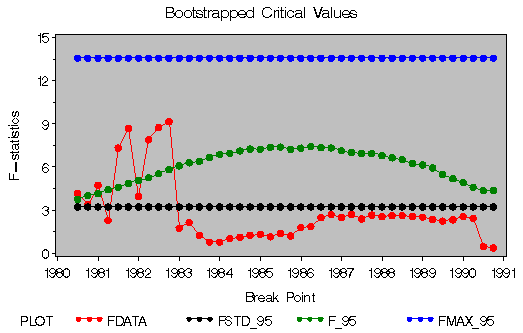Figure 2: Bootstrapped Critical Values

# References

Christiano, L. J. (1988), "Searching for a Break in GNP," N.B.E.R. Working Paper No. 2695.

Rappoport, P. and Reichlin, L. (1988), "Segmented Trends and Nonstationary Time Series," Economic Journal, 168-177.

SAS Institute Inc. (1990), SAS/IML Usage and Reference, Version 6, First Edition, Cary, NC: SAS Institute Inc.